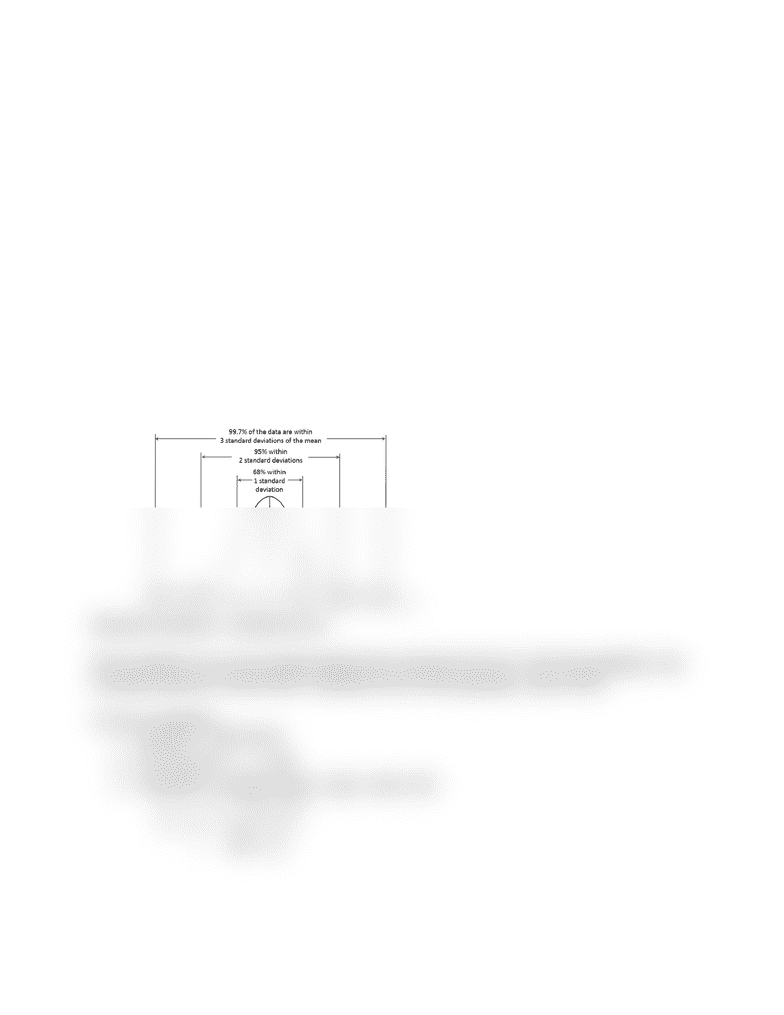Textbook Notes (280,000)
US (110,000)
KSU (300)
MATH (70)
Chapter 7.3 & 7.4

# MATH 10041 Chapter 7.3 & 7.4: MATH 10041-002 ch 7.3 CLT for sample proportions & 7.4 estimating the population proportion with confidence intervals

Department
Mathematics
Course Code
MATH 10041
Professor
Rajeev Rajaram
Chapter
7.3 & 7.4

This preview shows half of the first page. to view the full 2 pages of the document.Week 9
Wednesday
10/24/18
Chapter 7 cont
7.3 Central Limit Theorem for Sample Proportions
Central limit theorem (CLT)- used to estimate proportions on a population
Without needing to use simulations
Enables us to measure the quality of our estimation efforts
Has several versions
Some basic conditions must be met
Random and independent
Large sample size
AND p0n 1 (1 ) 0np≥ 1
Big population (N)
0nN ≥ 1
Recall the empirical rule (ch. 3.2)
***
Standard deviations = standard errors
If the conditions of a survey sample satisfy those required by the CLT, then the probability that
a sample proportion will fall within 2 standard errors of the population value is 95%
To find a probability…
1. Find SE =
p (1 ) / np
2. Find Z-score = SE/pp
3. 2ndVARS- normalcdf:-(lower, upper, mean, SE)
a. ( ),p,mean,SE 1
b. ( ),1,mean,SEp
i. SE = pσ